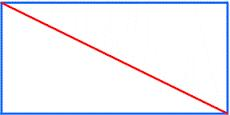# Centimeters 4162

Calculate for me the length of the diagonal of a rectangle whose size is 7 cm greater than its width and whose perimeter is 34 centimeters. The dimensions of the rectangle are expressed in natural numbers.

u =  13 cm

### Step-by-step explanation:Did you find an error or inaccuracy? Feel free to write us. Thank you!

Tips for related online calculators
Do you have a linear equation or system of equations and looking for its solution? Or do you have a quadratic equation?